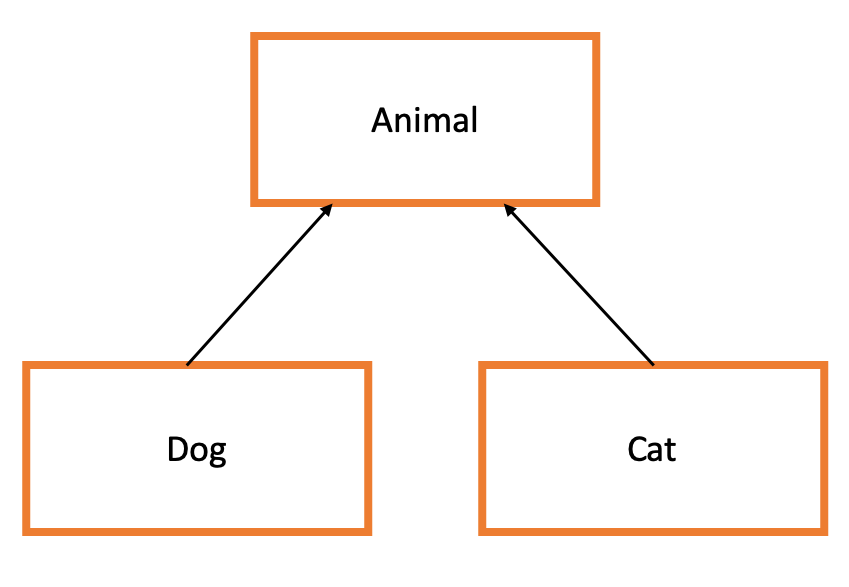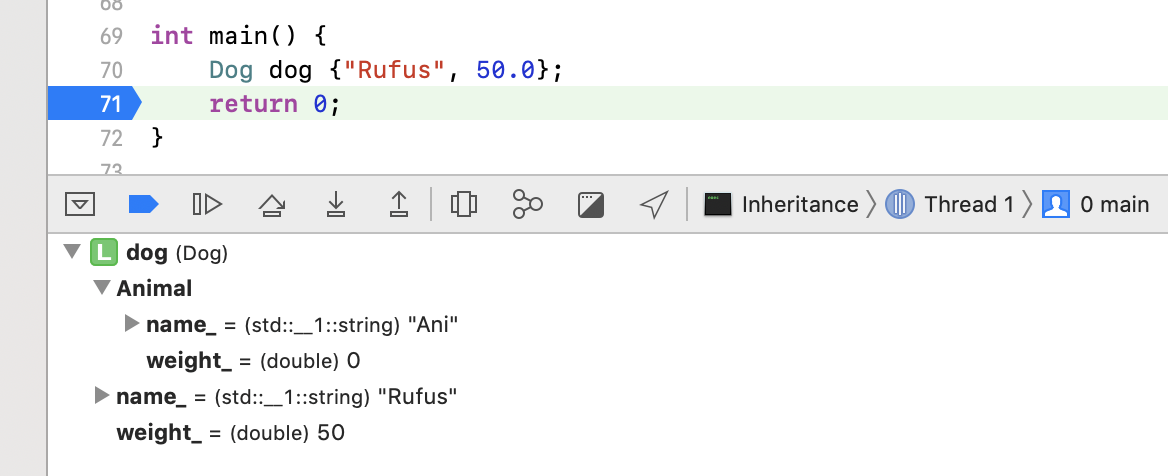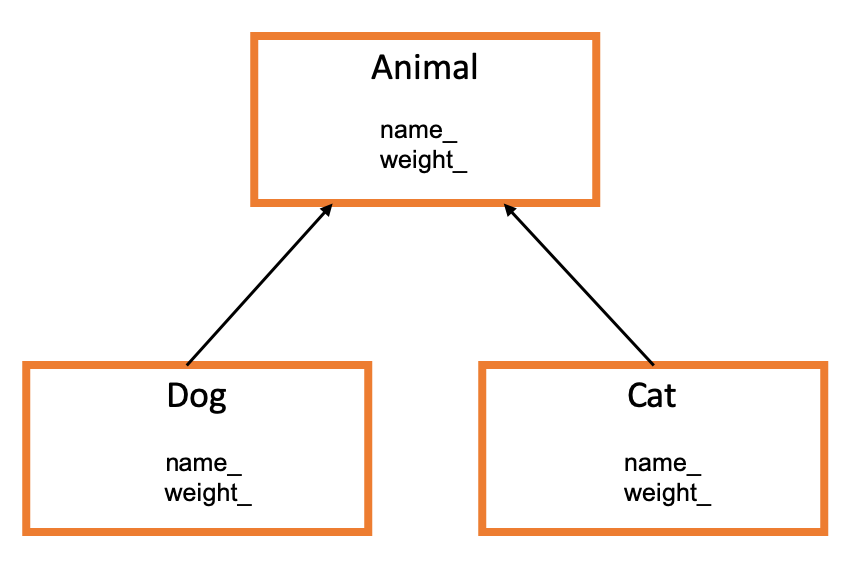# Introduction To Inheritance In C++

Contents

## Setup

In this tutorial, we’ll learn how inheritance works in C++ by tinkering with an example. Let’s start by building simple Dog and Cat classes.

### Dog Class

Create a Dog class that

1. Has member variables:
1. `string name_`
2. `double weight_`
2. Has member functions:
1. `void bark()` that prints “woof woof” to the console
2. `double top_speed()` that returns the dog’s top speed based on its weight
3. Has a `Dog(string name, double weight)` constructor that prints “Dog Constructor” to the console
``````#include <iostream>
#include <string>

class Dog {
public:

// Constructor
Dog(std::string name, double weight): name_{name}, weight_{weight} {
std::cout << "Dog Constructor" << std::endl;
};

// Getters
std::string get_name() { return name_; };
double get_weight() { return weight_; };

// Methods
void bark() { std::cout << "woof woof" << std::endl; };
double top_speed() { return (weight_ < 40) ? 15.5 : (weight_ < 90) ? 17.0 : 16.2; }

private:

// Member vars
std::string name_;
double weight_;
};
``````

### Cat Class

Create a Cat class that

1. Has member variables:
1. `string name_`
2. `double weight_`
2. Has member functions:
1. `void meow()` that prints “meowwww” to the console
2. `double top_speed()` that returns the cat’s top speed based on its weight
3. Has a `Cat(string name, double weight)` constructor that prints “Cat Constructor” to the console.
``````#include <iostream>
#include <string>

class Dog {...}

class Cat {
public:

// Constructor
Cat(std::string name, double weight): name_{name}, weight_{weight} {
std::cout << "Cat Constructor" << std::endl;
};

// Getters
std::string get_name() { return name_; };
double get_weight() { return weight_; };

// Methods
void meow() { std::cout << "meowwww" << std::endl; };
double top_speed() { return (weight_ < 10) ? 14.5 : (weight_ < 15) ? 16.2 : 12.9; }

private:

// Member vars
std::string name_;
double weight_;
};
``````

### Animal Class

You probably saw this one coming. Our Dog and Cat classes are very simlar, and as a result we’ve duplicated a lot of code. Let’s consolidate some things into a base class called Animal from which Dog and Cat can be derived. For now, we’ll keep it extremely simple, consiting only of a no-args constructor that prints “Animal Constructor” to the console.

``````#include <iostream>
#include <string>

class Dog {...}
class Cat {...}

class Animal {
public:

// Constructor
Animal() { std::cout << "Animal Constructor" << std::endl; };
};
``````

## Inheritance

We’ll use the following inheritance diagram to guide our design.In words, this means that a Dog is an Animal and a cat is an Animal. Let’s implement this by modifying our class declarations

`class Dog {``class Dog : public Animal {`
`class Cat {``class Cat : public Animal {`

The public keyword in that syntax is called an access specifier. Ignore it for now - we’ll discuss it later. Also, you probably need to re-organize your code so that Animal is declared before Dog and Cat, like so

``````#include <iostream>
#include <string>

class Animal {...}
class Dog : public Animal {...}
class Cat : public Animal {...}
``````

### Initialization

Now let’s see what happens when we initialize a new Dog instance.

``````int main() {
Dog dog {"Rufus", 50.0};
return 0;
}
``````

Animal Constructor
Dog Constructor

This illustrates an important point - when we create a new Dog instance, both the `Animal()` constructor and the `Dog(std::string name, double weight)` constructor are called, and they’re called in that order.

It makes sense for every animal to have a name and weight, so let’s 1) add name_ and weight_ member variables to our Animal class and 2) initialize them with dummy values in our `Animal()` constructor.

``````class Animal {
public:

// Constructor
Animal(): name_{"Ani"}, weight_{0.0} {
std::cout << "Animal Constructor" << std::endl;
};

private:

// Member vars
std::string name_;
double weight_;
};
``````

Once again, let’s instantiate a new Dog instance. However, this time we’ll set a break point just after dog gets instantiated so we can go under the hood and see what it looks like. (I’m doing this in Xcode.)This result may surprise you. Our Dog class’s name_ and weight_ variables are not overriding the Animal class’s name_ and weight_ variables. Instead, our dog instance is creating and holding on to 4 variables: name_ and weight_ scoped by Animal and a different name_ and weight_ scoped by Dog.#### Scopes

Since our dog object has two different version of name_ and weight_, it’s not obvious which versions will be returned by `get_name()` and `get_weight()`. Let’s check…

``````int main() {
Dog dog {"Rufus", 50.0};
std::cout << dog.get_name() << std::endl;
std::cout << dog.get_weight() << std::endl;
return 0;
}
``````

Animal Constructor
Dog Constructor
Rufus
50

So, within the derived class, name_ and weight_ refer to the derived class’s members. Suppose we wanted our Dog’s `get_name()` method to return the base class’s name_. We can achieve this by scoping the name_ variable with `Animal::name_` like so

``````class Dog : public Animal {
public:

// Constructor
Dog(std::string name, double weight): name_{name}, weight_{weight} {
std::cout << "Dog Constructor" << std::endl;
};

// Getters
std::string get_name() { return Animal::name_; };  // Return Animal's name_
double get_weight() { return weight_; };           // Return Dog's weight_

// Methods
void bark() { std::cout << "woof woof" << std::endl; };
double top_speed() { return (weight_ < 40) ? 15.5 : (weight_ < 90) ? 17.0 : 16.2; }

private:

// Member vars
std::string name_;
double weight_;
};
``````

Unfortunely this code doesn’t compile. The specific error that raises is ‘name_’ is a private member of ‘Animal’ - pretty telling.

#### Access Specifiers

Indeed name_ is a private member of Animal - therefore we cannot access it outside of the Animal class. Now you might be inclined to change name_ from private to public, but that solution is unideal. After all, we made name_ private for a reason - we don’t want people accessing and modifying it directly. C++ provides a nice solution to this problem - a 3rd access specifier called protected. If we declare Animal’s member variables as protected, they’ll be accessible from the Animal class and any class that inherits Animal. This is exactly what we want.

After making this change,

``````class Animal {
public:

// Constructor
Animal(): name_{"Ani"}, weight_{0.0} {
std::cout << "Animal Constructor" << std::endl;
};

protected: // Accessible by Animal and classes that inherit it

// Member vars
std::string name_;
double weight_;
};
``````

our Dog’s `std::string get_name() { return Animal::name_; };` method compiles and we get

``````int main() {
Dog dog {"Rufus", 50.0};
std::cout << dog.get_name() << std::endl;
std::cout << dog.get_weight() << std::endl;
return 0;
}
``````

Animal Constructor
Dog Constructor
Ani
50

Now it’s time to circle back. Remember when we declared `class Dog : public Animal {...`? That “public” is also an access specifer and it determines whether Animal’s members should stay the same or become less accessible once they get inherited by Dog. Alok Save has a good explanation of this on Stack Overflow. Here’s the gist of it, using our Animal example.

1. Every private member of Animal will only be accessible within Animal
2. Private Inheritance: `class Dog : private Animal {...`
1. protected members of Animal become private within Dog
2. public members of Animal become private within Dog
3. Protected Inheritance: `class Dog : protected Animal {...`
1. protected members of Animal remain protected within Dog
2. public members of Animal become protected within Dog
4. Public Inheritance: `class Dog : public Animal {...`
1. protected members of Animal remain protected within Dog
2. public members of Animal remain public within Dog

#### Constructors

Let’s get back to the problem at hand. We want every Animal to have a single name and weight. In this case, it makes sense to remove the name_ and weight_ member variables from our Dog and Cat classes, and only maintain them in the Animal class. Since we want Dog and Cat to have access to those variables, we’ll make them protected in the Animal class (as opposed to private). Furthermore, we should move the `get_name()` and `get_weight()` methods out of the derived classes and into the base class.

``````class Animal {
public:

// Constructor
Animal(): name_{"Ani"}, weight_{0.0} {
std::cout << "Animal Constructor" << std::endl;
};

// Getters
std::string get_name() { return name_; };
double get_weight() { return weight_; };

protected:

// Member vars
std::string name_;
double weight_;
};

class Dog : public Animal {
public:

// Constructor
Dog(std::string name, double weight): name_{name}, weight_{weight} {
std::cout << "Dog Constructor" << std::endl;
};

// Methods
void bark() { std::cout << "woof woof" << std::endl; };
double top_speed() { return (weight_ < 40) ? 15.5 : (weight_ < 90) ? 17.0 : 16.2; }
};

// Similar adjustments to Cat class not shown
``````

Close but no cigar; the above code does not compile. The culprit is our Dog constructor, `Dog(std::string name, double weight): name_{name}, weight_{weight} ...`. The compiler complains that name_ and weight_ are not members of Dog. Fair enough. What we want to do is use the parameters in the Dog constructor to initialize the inherited Animal methods. To do this, we need to make the appropriate Animal constructor and then we can invoke it within our Dog constructor.

``````class Animal {
public:

// Constructor
Animal(std::string name, double weight): name_{name}, weight_{weight} {
std::cout << "Animal Constructor" << std::endl;
};

// Getters
std::string get_name() { return name_; };
double get_weight() { return weight_; };

protected: // Accessible by Animal and classes that inherit it

// Member vars
std::string name_;
double weight_;
};

class Dog : public Animal {
public:

// Constructor (invokes the Animal(string name, double weight) constructor)
Dog(std::string name, double weight): Animal{name, weight} {
std::cout << "Dog Constructor" << std::endl;
}

// Methods
void bark() { std::cout << "woof woof" << std::endl; };
double top_speed() { return (weight_ < 40) ? 15.5 : (weight_ < 90) ? 17.0 : 16.2; }
};

// Similar adjustments to Cat class not shown
``````

Alas our compiler is happy and our inheritance design is looking better. Let’s give it a test.

``````int main() {
Dog dog {"Rufus", 50.0};
std::cout << dog.get_name() << std::endl;
std::cout << dog.get_weight() << std::endl;
return 0;
}
``````

Animal Constructor
Dog Constructor
Rufus
50

👍 This is working as desired, but there’s still a nagging issue. As it stands, someone could instantiate an generic Animal (i.e. not a dog or cat) with something like `Animal ryan{"Ryan", 100.0}`; We don’t want this. To prevent it, we can make the Animal constructor protected instead of public. Then it can still be called by a derived class but not otherwise.

``````class Animal {
public:

// Getters
std::string get_name() { return name_; };
double get_weight() { return weight_; };

protected: // Accessible by Animal and classes that inherit it

// Constructor
Animal(std::string name, double weight): name_{name}, weight_{weight} {
std::cout << "Animal Constructor" << std::endl;
};

// Member vars
std::string name_;
double weight_;
};
``````

Now if we try to make a new Animal inside main() or something we’ll get a compiler error.

### Virtual Functions

Suppose we want to make a global function, `bool animal_is_faster(Animal& a1, Animal& a2)` that determines if one animal is faster than another. We implement it like so

``````// Returns true if a1's top speed is > a2's top speed
bool animal_is_faster(Animal& a1, Animal& a2){
return a1.top_speed() > a2.top_speed();
}
``````

This produces a compiler error, No member named ‘top_speed’ in ‘Animal’. Even though Cat and Dog each have a `top_speed()` method, there’s no guarantee that they do. Furthermore, there’s no guarantee that a new Animal subclass will have a `top_speed()` method.

#### Pure Virtual Functions

We can solve this by creating a “pure virtual function” in our Animal class.

``````class Animal {
public:

// Getters
// ...

// Methods
// pure virtual function - to be implemented by derived class
virtual double top_speed() = 0;

protected: // Accessible by Animal and classes that inherit it

// Constructor
// ...

// Member vars
// ...
};
``````

This pure virtual function is basically saying “any class that inherits Animal must implement the method `double top_speed()`”. With this guarantee in place, our compiler lets us declare and use the method `bool animal_is_faster(Animal& a1, Animal& a2)` we implemented earlier. When we use Animal in this way, we call it an abstract base class.

Definition: A class with at least one pure virtual functions is an abstract class.

#### (Non Pure) Virtual Functions

If we could devise some sort of reasonable default implementation for an Animal’s `top_speed()` method, we could change it from a pure virtual function to a plain ole virtual function. Here’s an example

``````class Animal {
public:

// Getters
// ...

// Methods
// virtual function - may be overriden by derived class
virtual double top_speed() { return weight_ / 10; };

protected:

// Constructor
// ...

// Member vars
// ...
};
``````

This virtual function is saying “any class that inherits Animal gets this default implementation for the method `double top_speed()`, but may override it.”

Within our Dog and Cat classes, we can explicitly tell the compiler (and the reader) what methods are being overriden by using the override identifier.

``````class Dog : public Animal {
public:

// Constructor (invokes the Animal(string name, double weight) constructor)
// ...

// Methods
void bark() { std::cout << "woof woof" << std::endl; };
double top_speed() override { return (weight_ < 40) ? 15.5 : (weight_ < 90) ? 17.0 : 16.2; }
};
``````

The override keyword is not necessary but it’s a good convention to pick up. If we make a typo like `top_sped() override`, our compiler will fuss Hey, you’re trying override a function called “top_sped()”" but that function doesn’t exist in the base class!

### Polymorphism and Object Slicing

Let’s revert our last change to the Animal class, making the `top_speed()` method a pure virtual function, thus making Animal an abstract base class.

``````class Animal {
public:

// Getters
// ....

// Methods
// pure virtual function - must be overriden by derived class
double virtual top_speed() = 0;

protected: // Accessible by Animal and classes that inherit it

// Constructor
// ...

// Member vars
// ...
};
``````

Now, let’s see what happens if we try to copy a Dog instance to a variable of type Animal.

``````int main() {
Dog rufus {"Rufus", 50.0};
Animal rufusCopy = rufus;  // Error: Variable type 'Animal' is an abstract base class
std::cout << "Top Speed: " << rufusCopy.top_speed() << std::endl;
return 0;
}
``````

We get a compiler error telling us that Animal is an abstrct base class. So, if you want to do something like this, you need to use a pointer.

``````int main() {
Dog rufus {"Rufus", 50.0};
Animal* rufusPtr = &rufus;
std::cout << "Top Speed: " << rufusPtr->top_speed() << std::endl;
return 0;
}
``````

Now let’s undo our changes to Animal, so that `top_speed()` has a default implementation, thus making Animal not an abstract base class.

``````class Animal {
public:

// Getters
// ....

// Methods
// virtual function - may be overriden by derived class
double virtual top_speed() { return weight_ / 10.0; };

protected: // Accessible by Animal and classes that inherit it

// Constructor
// ...

// Member vars
// ...
};
``````

Now if we try to copy a Dog instance to a variable of type Animal, it works.

``````int main() {
Dog rufus {"Rufus", 50.0};
Animal rufusCopy = rufus;
return 0;
}
``````

The first time I saw this, I was scratching my head for the following reason. Suppose I declare a vector of Animals and reserve space for two of them. Then I could assign a Dog to the first element and a Cat to the second element. But suppose my Dog class has more member variables than my Cat class, and thus it requires more memory. How does `std::vector` know how much space to pre-allocate? It doesn’t know if I’m going to fill it with two dogs (a lot of memory) or two cats (a little bit of memory)?

The answer is object slicing. When we copy a Dog instance to a variable of type Animal, we don’t get all the extra stuff specific to our Dog class; we only get the stuff defined in Animal.

Related is the concept of polymorphism. If we copy a Dog instance to a variable of type Animal, then the copied object will be associated with Animal’s methods, not Dog’s.

``````// Example 1
int main() {
Dog rufus {"Rufus", 50.0};
Animal rufusCopy = rufus;

rufus.bark();
rufusCopy.bark();  // Error: No member named 'bark' in Animal

return 0;
}

// Example 2
int main() {
Dog rufus {"Rufus", 50.0};
Animal rufusCopy = rufus;

std::cout << rufus.top_speed() << std::endl;     //  17
std::cout << rufusCopy.top_speed() << std::endl; //  5

return 0;
}
``````

In closing, Inheritance is a powerful feature of C++ but that power comes at the cost of complexity. Only use it when the problem screams for it.